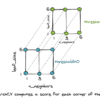# Author Archive | Mehreen Saeed## A Gentle Introduction To Vector Valued Functions

Vector valued functions are often encountered in machine learning, computer graphics and computer vision algorithms. They are particularly useful for defining the parametric equations of space curves. It is important to gain a basic understanding of vector valued functions to grasp more complex concepts. In this tutorial, you will discover what vector valued functions are, […]## A Gentle Introduction to Continuous Functions

Many areas of calculus require an understanding of continuous functions. The characteristics of continuous functions, and the study of points of discontinuity are of great interest to the mathematical community. Because of their important properties, continuous functions have practical applications in machine learning algorithms and optimization methods. In this tutorial, you will discover what continuous […]## A Gentle Introduction to Indeterminate Forms and L’Hospital’s Rule

Indeterminate forms are often encountered when evaluating limits of functions, and limits in turn play an important role in mathematics and calculus. They are essential for learning about derivatives, gradients, Hessians, and a lot more. In this tutorial, you will discover how to evaluate the limits of indeterminate forms and the L’Hospital’s rule for solving […]## A Gentle Introduction to Slopes and Tangents

The slope of a line, and its relationship to the tangent line of a curve is a fundamental concept in calculus. It is important for a general understanding of function derivatives. In this tutorial, you will discover what is the slope of a line and what is a tangent to a curve. After completing this […]## A Gentle Introduction to Derivatives of Powers and Polynomials

One of the most frequently used functions in machine learning and data science algorithms are polynomials or functions involving powers of x. It is therefore, important to understand how the derivatives of such functions are calculated. In this tutorial, you will discover how to compute the derivative of powers of x and polynomials. After completing […]## A Gentle Introduction to Function Derivatives

The concept of the derivative is the building block of many topics of calculus. It is important for understanding integrals, gradients, Hessians, and much more. In this tutorial, you will discover the definition of a derivative, its notation and how you can compute the derivative based upon this definition. You will also discover why the […]## A Gentle Introduction to Evaluating Limits

The concept of the limit of a function dates back to Greek scholars such as Eudoxus and Archimedes. While they never formally defined limits, many of their calculations were based upon this concept. Isaac Newton formally defined the notion of a limit and Cauchy refined this idea. Limits form the basis of calculus, which in […]## A Gentle Introduction to Limits and Continuity

There is no denying that calculus is a difficult subject. However, if you learn the fundamentals, you will not only be able to grasp the more complex concepts but also find them fascinating. To understand machine learning algorithms, you need to understand concepts such as gradient of a function, Hessians of a matrix, and optimization, […]## Modeling Pipeline Optimization With scikit-learn

This tutorial presents two essential concepts in data science and automated learning. One is the machine learning pipeline, and the second is its optimization. These two principles are the key to implementing any successful intelligent system based on machine learning. A machine learning pipeline can be created by putting together a sequence of steps involved […]